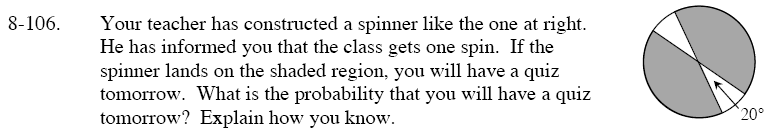Home > GC > Chapter 8 > Lesson 8.3.3 > Problem8-106

8-106.

Your teacher has constructed a spinner like the one at right. He has informed you that the class gets one spin. If the spinner lands on the shaded region, you will have a quiz tomorrow. What is the probability that you will have a quiz tomorrow? Explain how you know. Homework Help ✎The measure of all the angles that share a vertex at the center of a circle must add to 360°.

Vertical angles - the angles formed on opposite sides of two intersecting lines - are congruent to one another.

$\frac{320\degree}{360\degree} \approx 0.89\%$